Forcing as a computational process, Cambridge, Februrary 2019This will be a talk for Set Theory in the United Kingdom (STUK 1), to be held in the other place, February 16, 2019.

Abstract. We investigate the senses in which set-theoretic forcing can be seen as a computational process on the models of set theory. Given an oracle for the atomic or elementary diagram of a model of set theory $\langle M,\in^M\rangle$, for example, we explain senses in which one may compute $M$-generic filters $G\subset P\in M$ and the corresponding forcing extensions $M[G]$. Meanwhile, no such computational process is functorial, for there must always be isomorphic alternative presentations of the same model of set theory $M$ that lead by the computational process to non-isomorphic forcing extensions $M[G]\not\cong M[G’]$. Indeed, there is no Borel function providing generic filters that is functorial in this sense.

This is joint work with Russell Miller and Kameryn Williams.The rearrangement and subseries numbers: how much convergence suffices for absolute convergence? Mathematics Colloquium, University of Münster, January 2019

This will be a talk for the Mathematics Colloquium at the University of Münster, January 10, 2019.Abstract. The Riemann rearrangement theorem asserts that a series $\sum_n a_n$ is absolutely convergent if and only if every rearrangement $\sum_n a_{p(n)}$ of it is convergent, and furthermore, any conditionally convergent series can be rearranged so as to converge to any desired extended real value. How many rearrangements $p$ suffice to test for absolute convergence in this way? The rearrangement number, a new cardinal characteristic of the continuum, is the smallest size of a family of permutations, such that whenever the convergence and value of a convergent series is invariant by all these permutations, then it is absolutely convergent. The subseries number is defined similarly, as the smallest number of subseries whose convergence suffices to test a series for absolute convergence. The exact values of the rearrangement and subseries numbers turns out to be independent of the axioms of set theory. In this talk, I shall place the rearrangement and subseries numbers into a discussion of cardinal characteristics of the continuum, including an elementary introduction to the continuum hypothesis and an account of Freiling’s axiom of symmetry.

This talk is based in part on joint work with Andreas Blass, Joerg Brendle, Will Brian, myself, Michael Hardy and Paul Larson.

• The rearrangement number.
• A. Blass, J. Brendle, W. Brian, J. D. Hamkins, M. Hardy, and P. B. Larson, “The rearrangement number,” ArXiv e-prints, 2016. (manuscript under review)
@ARTICLE{BlassBrendleBrianHamkinsHardyLarson:TheRearrangementNumber,
author = {Andreas Blass and Jörg Brendle and Will Brian and Joel David Hamkins and Michael Hardy and Paul B. Larson},
title = {The rearrangement number},
journal = {ArXiv e-prints},
year = {2016},
volume = {},
number = {},
pages = {},
month = {},
note = {manuscript under review},
url = {http://jdh.hamkins.org/the-rearrangement-number},
eprint = {1612.07830},
archivePrefix = {arXiv},
primaryClass = {math.LO},
abstract = {},
keywords = {under-review},
source = {},
}

• The subseries number.

An infinitary-logic-free proof of the Barwise end-extension theorem, with new applications, University of Münster, January 2019

This will be a talk for the Logic Oberseminar at the University of Münster, January 11, 2019.Abstract. I shall present a new proof, with new applications, of the amazing extension theorem of Barwise (1971), which shows that every countable model of ZF has an end-extension to a model of ZFC + V=L. This theorem is both (i) a technical culmination of Barwise’s pioneering methods in admissible set theory and the admissible cover, but also (ii) one of those rare mathematical results saturated with significance for the philosophy of set theory. The new proof uses only classical methods of descriptive set theory, and makes no mention of infinitary logic. The results are directly connected with recent advances on the universal $\Sigma_1$-definable finite set, a set-theoretic version of Woodin’s universal algorithm.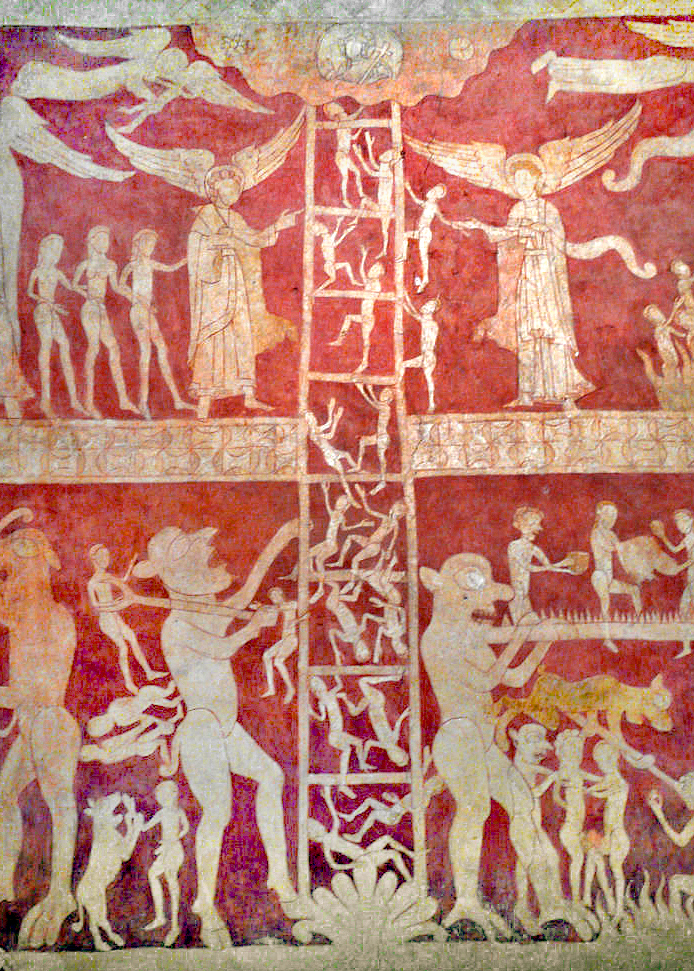A new proof of the Barwise extension theorem, without infinitary logic, CUNY Logic Workshop, December 2018

I’ll be back in New York from Oxford, and this will be a talk for the CUNY Logic Workshop, December 14, 2018.Abstract. I shall present a new proof, with new applications, of the amazing extension theorem of Barwise (1971), which shows that every countable model of ZF has an end-extension to a model of ZFC + V=L. This theorem is both (i) a technical culmination of Barwise’s pioneering methods in admissible set theory and the admissible cover, but also (ii) one of those rare mathematical results saturated with significance for the philosophy of set theory. The new proof uses only classical methods of descriptive set theory, and makes no mention of infinitary logic. The results are directly connected with recent advances on the universal $\Sigma_1$-definable finite set, a set-theoretic version of Woodin’s universal algorithm.

Faculty respondent to paper of Ethan Jerzak on Paradoxical Desires, Oxford Graduate Philosophy Conference, November 2018

The Oxford Graduate Philosophy Conference will be held at the Faculty of Philosophy November 10-11, 2018, with graduate students from all over the world speaking on their papers, with responses and commentary by Oxford faculty.I shall be the faculty respondent to the delightful paper, “Paradoxical Desires,” by Ethan Jerzak of the University of California at Berkeley, offered under the following abstract.

Ethan Jerzak (UC Berkeley): Paradoxical Desires
I present a paradoxical combination of desires. I show why it’s paradoxical, and consider ways of responding to it. The paradox saddles us with an unappealing disjunction: either we reject the possibility of the case by placing surprising restrictions on what we can desire, or we revise some bit of classical logic. I argue that denying the possibility of the case is unmotivated on any reasonable way of thinking about propositional attitudes. So the best response is a non-classical one, according to which certain desires are neither determinately satisfied nor determinately not satisfied. Thus, theorizing about paradoxical propositional attitudes helps constrain the space of possibilities for adequate solutions to semantic paradoxes more generally.

The conference starts with coffee at 9:00 am.  This session runs 11 am to 1:30 pm on Saturday 10 November in the Lecture Room.

Here are the notes I used for my response.Alan Turing, On computable numbers

I have been reading Alan Turing’s paper, On computable numbers, with an application to the entsheidungsproblem, an amazing classic, written by Turing while he was a student in Cambridge. This is the paper in which Turing introduces and defines his Turing machine concept, deriving it from a philosophical analysis of what it is that a human computer is doing when carrying out a computational task.

The paper is an incredible achievement. He accomplishes so much: he defines and explains the machines; he proves that there is a universal Turing machine; he shows that there can be no computable procedure for determining the validities of a sufficiently powerful formal proof system; he shows that the halting problem is not computably decidable; he argues that his machine concept captures our intuitive notion of computability; and he develops the theory of computable real numbers.What I was extremely surprised to find, however, and what I want to tell you about today, is that despite the title of the article, Turing adopts an incorrect approach to the theory of computable numbers. His central definition is what is now usually regarded as a mistaken way to proceed with this concept.

Let me explain. Turing defines that a computable real number is one whose decimal (or binary) expansion can be enumerated by a finite procedure, by what we now call a Turing machine. You can see this in the very first sentence of his paper, and he elaborates on and confirms this definition in detail later on in the paper.

He subsequently develops the theory of computable functions of computable real numbers, where one considers computable functions defined on these computable numbers. The computable functions are defined not on the reals themselves, however, but on the programs that enumerate the digits of those reals. Thus, for the role they play in Turing’s theory, a computable real number is not actually regarded as a real number as such, but as a program for enumerating the digits of a real number. In other words, to have a computable real number in Turing’s theory is to have a program for enumerating the digits of a real number. And it is this aspect of Turing’s conception of computable real numbers where his approach becomes problematic.

One specific problem with Turing’s approach is that on this account, it turns out that the operations of addition and multiplication for computable real numbers are not computable operations. Of course this is not what we want.

The basic mathematical fact in play is that the digits of a sum of two real numbers $a+b$ is not a continuous function of the digits of $a$ and $b$ separately; in some cases, one cannot say with certainty the initial digits of $a+b$, knowing only finitely many digits, as many as desired, of $a$ and $b$.

To see this, consider the following sum $a+b$
\begin{align*} &0.343434343434\cdots \\ +\quad &0.656565656565\cdots \\[-7pt] &\hskip-.5cm\rule{2in}{.4pt}\\ &0.999999999999\cdots \end{align*}
If you add up the numbers digit-wise, you get $9$ in every place. That much is fine, and of course we should accept either $0.9999\cdots$ or $1.0000\cdots$ as correct answers for $a+b$ in this instance, since those are both legitimate decimal representations of the number $1$.

The problem, I claim, is that we cannot assign the digits of $a+b$ in a way that will depend only on finitely many digits each of $a$ and $b$. The basic problem is that if we inspect only finitely many digits of $a$ and $b$, then we cannot be sure whether that pattern will continue, whether there will eventually be a carry or not, and depending on how the digits proceed, the initial digits of $a+b$ can be affected.

In detail, suppose that we have committed to the idea that the initial digits of $a+b$ are $0.999$, on the basis of sufficiently many digits of $a$ and $b$. Let $a’$ and $b’$ be numbers that agree with $a$ and $b$ on those finite parts of $a$ and $b$, but afterwards have all $7$s. In this case, the sum $a’+b’$ will involve a carry, which will turn all the nines up to that point to $0$, with a leading $1$, making $a’+b’$ strictly great than $1$ and having decimal representation $1.000\cdots00005555\cdots$. Thus, the initial-digits answer $0.999$ would be wrong for $a’+b’$, even though $a’$ and $b’$ agreed with $a$ and $b$ on the sufficiently many digits supposedly justifying the $0.999$ answer. On the other hand, if we had committed ourselves to $1.000$ for $a+b$, on the basis of finite parts of $a$ and $b$ separately, then let $a”$ and $b”$ be all $2$s beyond that finite part, in which case $a”+b”$ is definitely less than $1$, making $1.000$ wrong.

Therefore, there is no algorithm to compute the digits of $a+b$ continuously from the digits of $a$ and $b$ separately. It follows that there can be no computable algorithm for computing the digits of $a+b$, given the programs that compute $a$ and $b$ separately, which is how Turing defines computable functions on the computable reals. (This consequence is a subtly different and stronger claim, but one can prove it using the Kleene recursion theorem. Namely, let $a=.343434\cdots$ and then consider the program to enumerate a number $b$, which will begin with $0.656565$ and keep repeating $65$ until it sees that the addition program has given the initial digits for $a+b$, and at this moment our program for $b$ will either switch to all $7$s or all $2$s in such a way so as to refute the result. The Kleene recursion theorem is used in order to know that indeed there is such a self-referential program enumerating $b$.)

One can make similar examples showing that multiplication and many other very simple functions are not computable, if one insists that a computable number is an algorithm enumerating the digits of the number.

So what is the right definition of computable number? Turing was right that in working with computable real numbers, we want to be working with the programs that compute them, rather than the reals themselves somehow. What is needed is a better way of saying that a given program computes a given real.

The right definition, widely used today, is that we want an algorithm not to compute exactly the digits of the number, but rather, to compute approximations to the number, as close as desired, with a known degree of accuracy. One can define a computable real number as a computable sequence of rational numbers, such that the $n^{th}$ number is within $1/2^n$ of the target number. This is equivalent to being able to compute rational intervals around the target real, of size less than any specified accuracy. And there are many other equivalent ways to do it. With this concept of computable real number, then the operations of addition, multiplication, and so on, all the familiar operations on the real numbers, will be computable.

But let me clear up a confusing point. Although I have claimed that Turing’s original definition of computable real number is incorrect, and I have explained how we usually define this concept today, the mathematical fact is that a real number $x$ has a computable presentation in Turing’s sense (we can compute the digits of $x$) if and only if it has a computable presentation in the contemporary sense (we can compute rational approximations to any specified accuracy). Thus, in terms of which real numbers we are talking about, the two approaches are extensionally the same.

Let me quickly prove this. If a real number $x$ is computable in Turing’s sense, so that we can compute the digits of $x$, then we can obviously compute rational approximations to any desired accuracy, simply by taking sufficiently many digits. And conversely, if a real number $x$ is computable in the contemporary sense, so we can compute rational approximations to any specified accuracy, then either it is itself a rational number, in which case we can certainly compute the digits of $x$, or else it is irrational, in which case for any specified digit place, we can wait until we have a rational approximation forcing it to one side or the other, and thereby come to know this digit. (Note: there are issues of intuitionistic logic occurring here, precisely because we cannot tell from the approximation algorithm itself which case we are in.) Note also that this argument works in any desired base.

So there is something of a philosophical problem here. The issue isn’t that Turing has misidentified particular reals as being computable or non-computable or has somehow got the computable reals wrong extensionally as a subset of the real numbers, since every particular real number has Turing’s kind of representation if and only if it has the approximation kind of representation. Rather, the problem is that because we want to deal with computable real numbers by working with the programs that represent them, Turing’s approach means that we cannot regard addition as a computable function on the computable reals. There is no computable procedure that when given two programs for enumerating the digits of real numbers $a$ and $b$ returns a program for enumerating the digits of the sum $a+b$. But if you use the contemporary rational-approximation representation of computable real number, then you can computably produce a program for the sum, given programs for the input reals. This is the sense in which Turing’s approach is wrong.

On set-theoretic mereology as a foundation of mathematics, Oxford Phil Math seminar, October 2018

This will be a talk for the Philosophy of Mathematics Seminar in Oxford, October 29, 2018, 4:30-6:30 in the Ryle Room of the Philosopher Centre.Abstract. In light of the comparative success of membership-based set theory in the foundations of mathematics, since the time of Cantor, Zermelo and Hilbert, it is natural to wonder whether one might find a similar success for set-theoretic mereology, based upon the set-theoretic inclusion relation $\subseteq$ rather than the element-of relation $\in$.  How well does set-theoretic mereological serve as a foundation of mathematics? Can we faithfully interpret the rest of mathematics in terms of the subset relation to the same extent that set theorists have argued (with whatever degree of success) that we may find faithful representations in terms of the membership relation? Basically, can we get by with merely $\subseteq$ in place of $\in$? Ultimately, I shall identify grounds supporting generally negative answers to these questions, concluding that set-theoretic mereology by itself cannot serve adequately as a foundational theory.

This is joint work with Makoto Kikuchi, and the talk is based on our joint articles:

The talk will also mention some related recent work with Ruizhi Yang (Shanghai).Slides

Parallels in universality between the universal algorithm and the universal finite set, Oxford Math Logic Seminar, October 2018

This will be a talk for the Logic Seminar in Oxford at the Mathematics Institute in the Andrew Wiles Building on October 9, 2018, at 4:00 pm, with tea at 3:30.Abstract. The universal algorithm is a Turing machine program $e$ that can in principle enumerate any finite sequence of numbers, if run in the right model of PA, and furthermore, can always enumerate any desired extension of that sequence in a suitable end-extension of that model. The universal finite set is a set-theoretic analogue, a locally verifiable definition that can in principle define any finite set, in the right model of set theory, and can always define any desired finite extension of that set in a suitable top-extension of that model. Recent work has uncovered a $\Sigma_1$-definable version that works with respect to end-extensions. I shall give an account of all three results, which have a parallel form, and describe applications to the model theory of arithmetic and set theory.

Slides

The rearrangement number: how many rearrangements of a series suffice to validate absolute convergence? Warwick Mathematics Colloquium, October 2018

This will be a talk for the Mathematics Colloquium at the University of Warwick, to be held October 19, 2018, 4:00 pm in Lecture Room B3.02 at the Mathematics Institute. I am given to understand that the talk will be followed by a wine and cheese reception.Abstract. The Riemann rearrangement theorem asserts that a series $\sum_n a_n$ is absolutely convergent if and only if every rearrangement $\sum_n a_{p(n)}$ of it is convergent, and furthermore, any conditionally convergent series can be rearranged so as to converge to any desired extended real value. How many rearrangements $p$ suffice to test for absolute convergence in this way? The rearrangement number, a new cardinal characteristic of the continuum, is the smallest size of a family of permutations, such that whenever the convergence and value of a convergent series is invariant by all these permutations, then it is absolutely convergent. The exact value of the rearrangement number turns out to be independent of the axioms of set theory. In this talk, I shall place the rearrangement number into a discussion of cardinal characteristics of the continuum, including an elementary introduction to the continuum hypothesis and an account of Freiling’s axiom of symmetry.

This talk is based in part on joint work with Andreas Blass, Will Brian, myself, Michael Hardy and Paul Larson.

• The rearrangement number.
• A. Blass, J. Brendle, W. Brian, J. D. Hamkins, M. Hardy, and P. B. Larson, “The rearrangement number,” ArXiv e-prints, 2016. (manuscript under review)
@ARTICLE{BlassBrendleBrianHamkinsHardyLarson:TheRearrangementNumber,
author = {Andreas Blass and Jörg Brendle and Will Brian and Joel David Hamkins and Michael Hardy and Paul B. Larson},
title = {The rearrangement number},
journal = {ArXiv e-prints},
year = {2016},
volume = {},
number = {},
pages = {},
month = {},
note = {manuscript under review},
url = {http://jdh.hamkins.org/the-rearrangement-number},
eprint = {1612.07830},
archivePrefix = {arXiv},
primaryClass = {math.LO},
abstract = {},
keywords = {under-review},
source = {},
}

• The subseries number.

The propagation of error in classical geometry constructions

I’d like to discuss the issue of error and error propagation in the constructions of classical geometry. How does error propagate in these constructions? How sensitive are the familiar classical constructions to small errors in the use of the straightedge or compass?

Let me illustrate what I have in mind by considering the classical construction of Apollonius of the perpendicular bisector of a line segment $AB$.  One forms two circles, centered at $A$ and $B$ respectively, each with radius $AB$.These arcs intersect at points $P$ and $Q$, respectively, which form the perpendicular bisector, meeting the original segment at the midpoint $C$.That is all fine and good, and one can easily prove that indeed $PQ$ is perpendicular to $AB$ and that $C$ is the midpoint of $AB$, as desired.

When carrying out such a construction in practice, however, there will inevitably be some small errors. We do not expect to implement it exactly, with infinite precision, but rather, we expect some small errors in the placement of the compass or straightedge, and perhaps these errors may accumulate and they propagate through the construction. What I would like to discuss is the sensitivity of this construction and the other classical constructions to these small errors.For example, suppose that we are given points $A$ and $B$. When we seek to construct the circle centered at $A$ with radius $AB$, we place the point of the compass at $A$, and this placement may have some small error deviating from $A$, landing somewhere in the blue circle. Similarly, the writing (or etching) implement end of the compass is placed at $B$, with its own possibly different error, landing the orange circle at $B$. The arc actually resulting will be some arc arising with some such small errors, like this: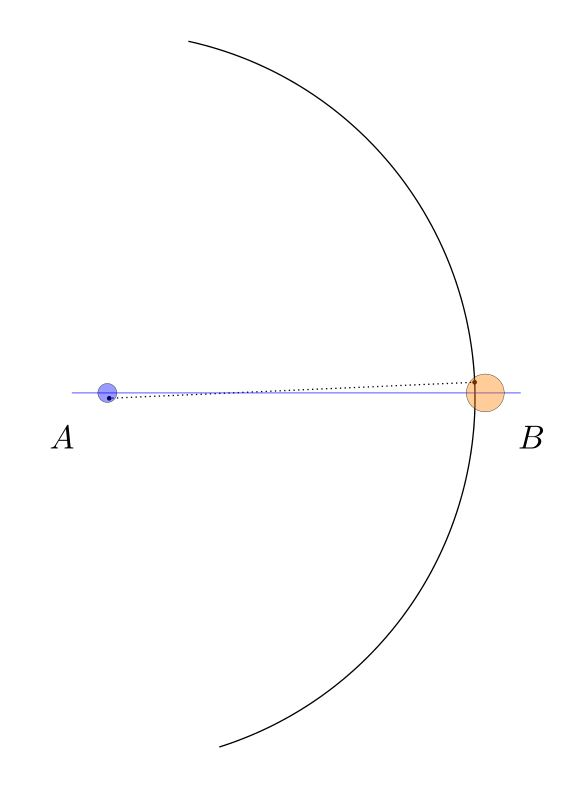We may represent the space of all arcs that could arise in conformance with those error bounds as the blurred orange arc below. This image was created simply by drawing many dozens of such arcs in orange, with various choices for the center and radius within the error circles, and blending the results together.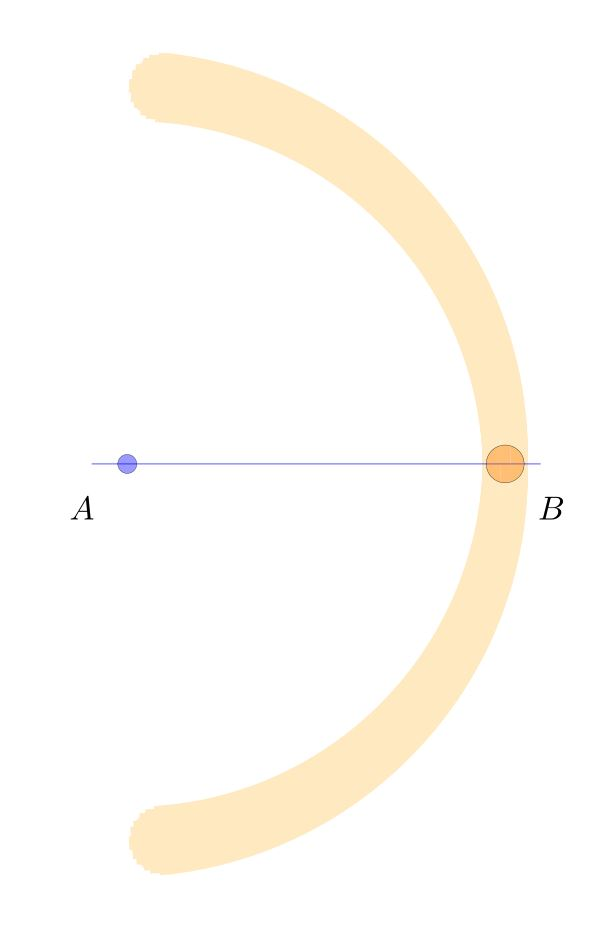We carry out the same construction with similar errors for the other arc, centered at $B$ and passing through $A$. These arcs overlap in the darker orange regions above and below, determining the points $P$ and $Q$.The actual arcs we draw and the corresponding vertical will land somewhere inside these blurred regions, perhaps like this:Note that in this particular case, the resulting line $PQ$ is noticably non-perpendicular to $AB$, and the resulting point $C$ is noticably not the midpoint. Consider the space of all the bisectors $PQ$ that might arise in conformance with our errors on $A$ and $B$, showing the result as the vertical red shaded region.The darker red region is the space of possible points $C$ that we might have constructed as the “midpoint” $C$, in conformance with the error estimates.

Given the size of the original error bounds on the points $A$ and $B$, it may be surprising that even such a standard simple construction as this — constructing the perpendicular bisector and midpoint of a segment — appears to have comparatively large error propagation, since the shaded red region $C$ is quite large and includes many points that one would not say are close to being the midpoint.  In this sense, the Apollonius perpendicular bisector construction appears to be sensitive to the errors of compass placement.

Is there a better construction? For example, in terms of improving the accuracy at least of the perpendicularity of $PQ$ to $AB$, it would seem to help to use a much larger circle, which would lower the variation in the resulting “right” angle.  But this is partly because we have so far assumed that compass error arises only with the placement of the points of the compass, and not during the course of actually drawing the arc. But of course, one can imagine that errors arise from a flexing of the compass during use, causing it to deviate from circularity, or from slippage, which might reasonably be expected to cause increasing error with the length or degree of the arc, and so on, and such a model of error might have greater errors with large circles.

One could in principle carry out similar analyses for any geometric construction, and use the corresponding results to compare the sensitivity of various methods for constructing the same object, as well as modeling different sources of error.  The goal might be to mount a precise analysis of all the standard constructions and compare competing constructions for accuracy.

There is a literature of papers doing precisely this, and I will try to post some references later (or please do so in the comments, if you have some good ones).

Another approach to error estimation would be to think of the errors at points $A$ and $B$ as probability distributions, centered at $A$ and $B$ and with a certain variation; and one then gets corresponding distributions for the points $P$ and $Q$, which are not rigid shapes as in my diagrams, but qualitatively similar distributions spread out in that region, and a resulting probability distribution for the point $C$.

Finally, let me mention that one might hope to improve the accuracy of a construction, simply by repeating it and averaging the result, or by some other convergence algorithm. For example, as a first step, we might simply perform the Apollonius bisector construction twice, producing midpoint candidates $C_0$ and $C_1$, and we could proceed simply find the midpoint of $C_0C_1$ as a further presumably more accurate midpoint. Or we could iterate in some other manner and hope to converge to the actual midpoint. For example, we could produce seven midpoint candidates, and take the resulting median point.

Draw an infinite chessboard in perspective, using straightedge only

I’d like to explain to you how to draw chessboards by hand in perfect perspective, using only a straightedge.  In this post, I’ll explain how to construct chessboards of any size, starting with the size of the basic unit square.This post follows up on the post I made yesterday about how to draw a chessboard in perspective view, using only a straightedge.  That method was a subdivision method, where one starts with the boundary of the desired board, and then subdivides to make a chessboard. Now, we start with the basic square and build up. This method is actually quite efficient for quickly making very large boards in perspective view.

I want to emphasize that this is something that you can actually do, right now. It’s fun! All you need is a piece of paper, a pencil and a straightedge. I’ll wait right here while you gather your materials. Use a ruler or a chop stick (as I did) or the edge of a notebook or the lid of a box. Sit at your table and draw a huge chessboard in perspective. You can totally do this.

Start with a horizon, having two points at infinity (orange), at left and right, and a third point midway between them (brown), which we will call the diagonal infinity. Also, mark the front corner of your chess board (blue).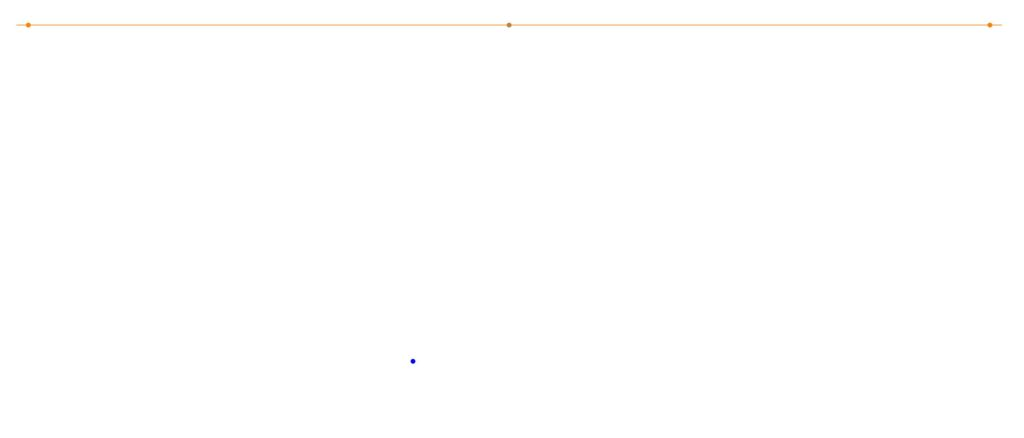Extend the front corner to the points at infinity. And then mark off (red) a point that will be a measure of the grid spacing in the chessboard. This will the be size of the front square.You can extend that point to infinity at the right. This delimits the first rank of the chessboard.Next, extend the front corner of the board to the diagonal infinity.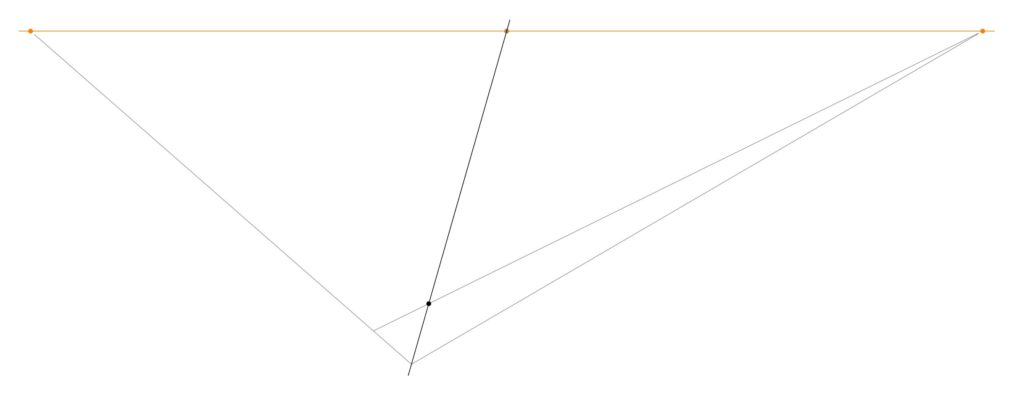The intersection of that diagonal with the previous line determines a point, which when extended to infinity at the left, produces the first square of the chessboard.And that line determines a new point on the leading rank edge. Extend that point up to the diagonal infinity, which determines another point on the second rank line.Extend that line to infinity at the left, which determines another point on the leading rank edge.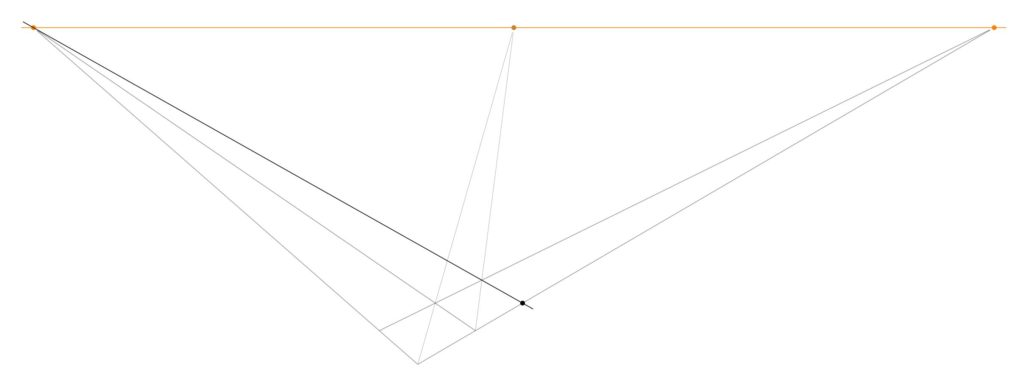Continuing in this way, one can produce as many first rank squares as desired. Go ahead and do that. At each step, you extend up to the diagonal infinity, which determines a new point, which when extended to infinity at the left determines another point, and so on.If you should now reflect on the current diagram, you may notice that we have actually determined many further points in the grid than we have mentioned — and thanks to my daughter Hypatia for noticing this simplification — for there is a whole triangle of further intersection points between the files and the diagonals.We can use these points (and we do not need them all) to construct the rest of the board, by drawing out the lines to infinity at the right. Thus, we construct the whole chessboard:One can construct a perspective chessboard of any size this way, and one can simply continue with the construction and make it larger, if desired.

It will look a little better if you add a point at infinity down below (and do so directly below the diagonal point at infinity, but a good distance down below the board), and extend the board downward one level. The corresponding diagram on yesterday’s post might be helpful.You can now color the tile pattern, and you’ll have a chessboard in perfect perspective view.If you keep going, you can make extremely large chessboards. In time, I hope that you will come to learn how to complete an infinite chess board in finite time.Draw a chessboard in perspective view, using straightedge onlyLet me show you how to hand-draw an image of a chessboard in perspective, using only a straightedge. There is no need to measure any distances or to make calculations of any kind.  All you need is a straightedge, paper and your pen or pencil.

Imagine the vast doodling potential!  A person who happens to be stuck in a lecture on some other less interesting topic could easily make one or more elaborate chessboards in perfect perspective, using only a straightedge! Please carry out the construction and then share your resulting images. I would love to see them.

To begin, give yourself a horizon at the top of the page, with two points at infinity (in orange), and also two points (blue) that will become the front and back corner of your chessboard.  You can play around with different arrangements of these points, which will lead ultimately to different perspective views.Using your straightedge, draw the lines from those reference points to the points at infinity. The resulting enclosed region will ultimately become the main chess board. One can alternatively think of starting with the front edges of the desired board, and then setting a horizon and using that to determine the points at infinity, and then adding the back edges.If you like, you can add a third point at infinity down below, and a front bottom reference point (blue), to give the board some thickness. Construct the lines to the bottom point at infinity.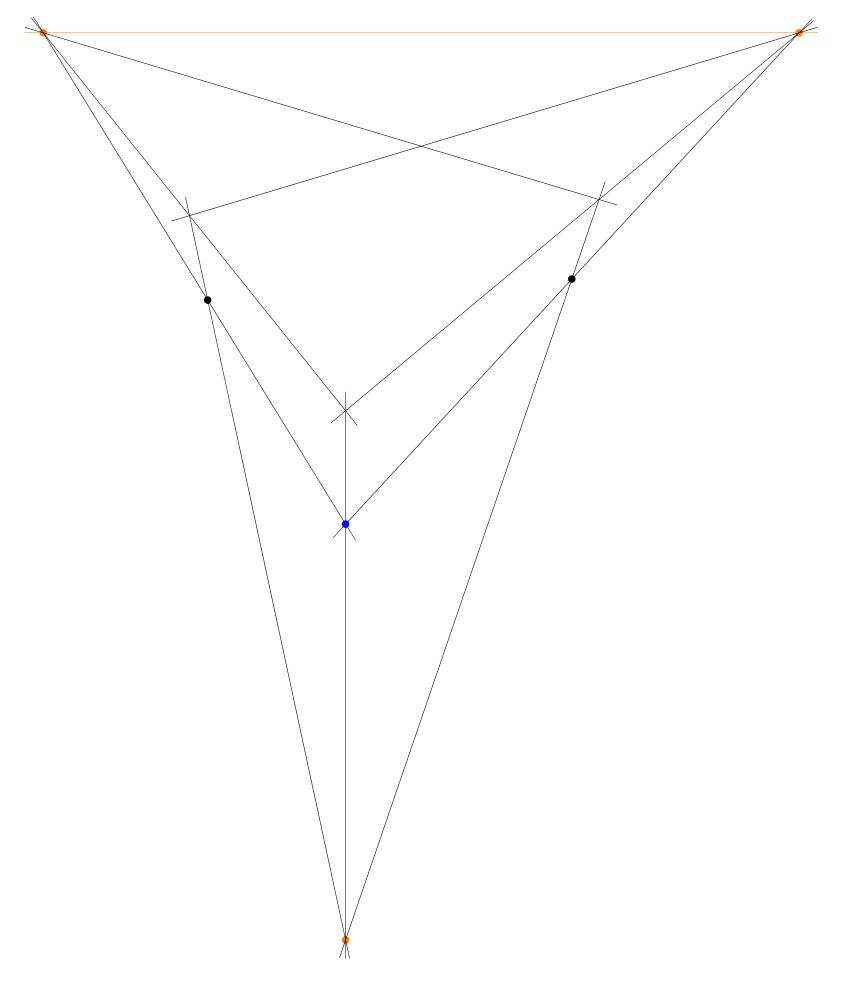The result is now the main outline of your chessboard as a rectangular solid.Next, construct the center point of the top face, by drawing the two diagonals and finding the intersection point. I call this the center point, because it is the point that represents in the perspective view the actual center point of the chessboard, even though this point is not in the “center” of the quadrilateral representing the board. It is remarkable that one can find that point without needing to measure or calculate anything, simply because the two straight lines of the diagonal of the chessboard intersect at that point, and this remains true in the perspective view. This is the key idea that enables the entire construction method to proceed with only a straightedge.Construct the midpoint lines by drawing the lines from that centerpoint to the points at infinity.Now you have the chessboard with the main midlines drawn.Construct the center points of the two diagonal squares, by intersecting the diagonals of each of them.Construct the lines that extend those points to the points at infinity.Thus, you have constructed the main 4 x 4 grid.You can extend the grid lines down to the bottom point at infinity like so:One can stop with the 4 x 4 board, if desired. Simply add suitable shading, and you’ve completed the 4 x 4 chessboard in perspective.Alternatively, one can continue with one more iteration to construct the 8 x 8 board. From the 4 x 4 grid (with no shading yet) simply construct the center points of the squares on the main diagonal, and extend those lines to infinity.  This will enable you to draw the 8 x 8 grid lines, and after shading, you’ll have the complete chessboard.The initial arrangement of points affects the nature of the perspective view. Having the points at infinity very far away will produce something closer to orthoprojection; having them close produces a more extreme perspective, which simulates a view from a vantage point extremely close to the board.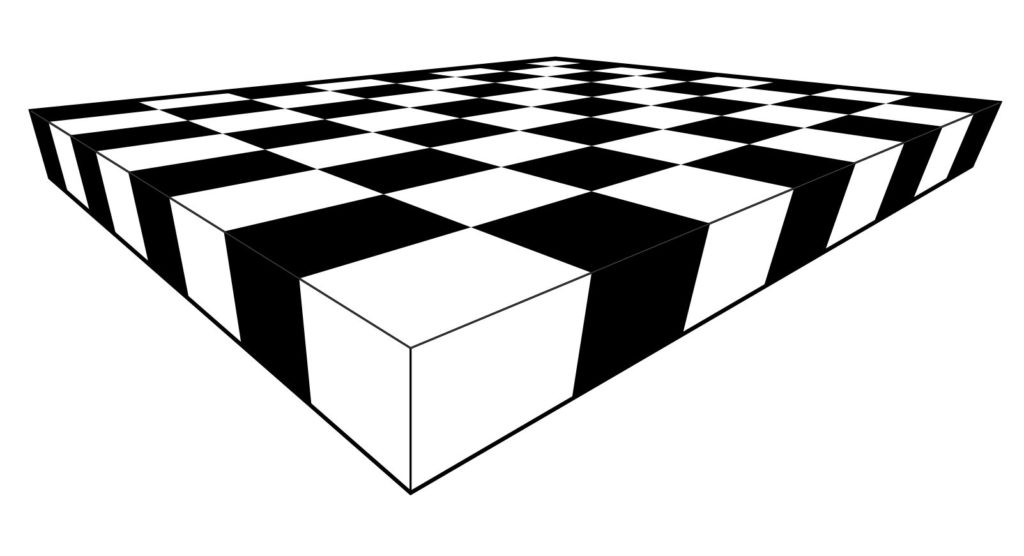Please give the construction a try! All you need is paper, pencil and a straightedge! Provide links below in the comments to photos of your creations.

Meanwhile, let me point you towards my follow post, How to draw infinite chessboards by hand in perfect perspective, using only a straightedge. The difference between the methods is that the method of this post is about subdividing a given board, and the other method is about generating arbitrarily large chessboards from a given unit square.

I learned these construction method years ago from my CUNY geometer colleague Ilya Kofman.

The axiom of well-ordered replacement is equivalent to full replacement over Zermelo + foundationIn recent work, Alfredo Roque Freire and I have realized that the axiom of well-ordered replacement is equivalent to the full replacement axiom, over the Zermelo set theory with foundation.

The well-ordered replacement axiom is the scheme asserting that if $I$ is well-ordered and every $i\in I$ has unique $y_i$ satisfying a property $\phi(i,y_i)$, then $\{y_i\mid i\in I\}$ is a set. In other words, the image of a well-ordered set under a first-order definable class function is a set.

Alfredo had introduced the theory Zermelo + foundation + well-ordered replacement, because he had noticed that it was this fragment of ZF that sufficed for an argument we were mounting in a joint project on bi-interpretation. At first, I had found the well-ordered replacement theory a bit awkward, because one can only apply the replacement axiom with well-orderable sets, and without the axiom of choice, it seemed that there were not enough of these to make ordinary set-theoretic arguments possible.

But now we know that in fact, the theory is equivalent to ZF.

Theorem. The axiom of well-ordered replacement is equivalent to full replacement over Zermelo set theory with foundation.

$$\text{ZF}\qquad = \qquad\text{Z} + \text{foundation} + \text{well-ordered replacement}$$

Proof. Assume Zermelo set theory with foundation and well-ordered replacement.

Well-ordered replacement is sufficient to prove that transfinite recursion along any well-order works as expected. One proves that every initial segment of the order admits a unique partial solution of the recursion up to that length, using well-ordered replacement to put them together at limits and overall.

Applying this, it follows that every set has a transitive closure, by iteratively defining $\cup^n x$ and taking the union. And once one has transitive closures, it follows that the foundation axiom can be taken either as the axiom of regularity or as the $\in$-induction scheme, since for any property $\phi$, if there is a set $x$ with $\neg\phi(x)$, then let $A$ be the set of elements $a$ in the transitive closure of $\{x\}$ with $\neg\phi(a)$; an $\in$-minimal element of $A$ is a set $a$ with $\neg\phi(a)$, but $\phi(b)$ for all $b\in a$.

Another application of transfinite recursion shows that the $V_\alpha$ hierarchy exists. Further, we claim that every set $x$ appears in the $V_\alpha$ hierarchy. This is not immediate and requires careful proof. We shall argue by $\in$-induction using foundation. Assume that every element $y\in x$ appears in some $V_\alpha$. Let $\alpha_y$ be least with $y\in V_{\alpha_y}$. The problem is that if $x$ is not well-orderable, we cannot seem to collect these various $\alpha_y$ into a set. Perhaps they are unbounded in the ordinals? No, they are not, by the following argument. Define an equivalence relation $y\sim y’$ iff $\alpha_y=\alpha_{y’}$. It follows that the quotient $x/\sim$ is well-orderable, and thus we can apply well-ordered replacement in order to know that $\{\alpha_y\mid y\in x\}$ exists as a set. The union of this set is an ordinal $\alpha$ with $x\subseteq V_\alpha$ and so $x\in V_{\alpha+1}$. So by $\in$-induction, every set appears in some $V_\alpha$.

The argument establishes the principle: for any set $x$ and any definable class function $F:x\to\text{Ord}$, the image $F\mathrel{\text{”}}x$ is a set. One proves this by defining an equivalence relation $y\sim y’\leftrightarrow F(y)=F(y’)$ and observing that $x/\sim$ is well-orderable.

We can now establish the collection axiom, using a similar idea. Suppose that $x$ is a set and every $y\in x$ has a witness $z$ with $\phi(y,z)$. Every such $z$ appears in some $V_\alpha$, and so we can map each $y\in x$ to the smallest $\alpha_y$ such that there is some $z\in V_{\alpha_y}$ with $\phi(y,z)$. By the observation of the previous paragraph, the set of $\alpha_y$ exists and so there is an ordinal $\alpha$ larger than all of them, and thus $V_\alpha$ serves as a collecting set for $x$ and $\phi$, verifying this instance of collection.

From collection and separation, we can deduce the replacement axiom $\Box$

I’ve realized that this allows me to improve an argument I had made some time ago, concerning Transfinite recursion as a fundamental principle. In that argument, I had proved that ZC + foundation + transfinite recursion is equivalent to ZFC, essentially by showing that the principle of transfinite recursion implies replacement for well-ordered sets. The new realization here is that we do not need the axiom of choice in that argument, since transfinite recursion implies well-ordered replacement, which gives us full replacement by the argument above.

Corollary. The principle of transfinite recursion is equivalent to the replacement axiom over Zermelo set theory with foundation.

$$\text{ZF}\qquad = \qquad\text{Z} + \text{foundation} + \text{transfinite recursion}$$

There is no need for the axiom of choice.

Lectures on the Philosophy of Mathematics, Oxford, Michaelmas 2018

This will be a series of lectures on the philosophy of mathematics, given at Oxford University, Michaelmas term 2018. The lectures are mainly intended for undergraduate students preparing for exam paper 122, although all interested parties are welcome.

My approach to the philosophy of mathematics tends to be grounded in mathematical arguments and ideas, treating philosophical issues as they arise organically. The lectures will accordingly be organized around mathematical themes, in such a way that naturally brings various philosophical issues to light.Here is a tentative list of topics, which may be updated as the term approaches.

Lecture 1. Numbers. Numbers are perhaps the essential mathematical idea, but what are numbers? We have many kinds of numbers—natural numbers, integers, rational numbers, real numbers, complex numbers, hyperreal numbers, surreal numbers, ordinal numbers, and more—and these number systems provide a fruitful background for classical arguments on incommensurability, the irrationality of $\sqrt{2}$, transcendental numbers, the infinitude of primes, and lead naturally to discussions of platonism, Frege’s number concept, Peano’s numbers, Dedekind’s categoricity arguments, and the philosophy of structuralism.

Lecture 2. Rigour. Let us consider the problem of mathematical rigour in the development of the calculus. Informal continuity concepts and the use of infinitesimals ultimately gave way to formal epsilon-delta limit concepts, which provided a capacity for refined notions, such as uniform continuity, equicontinuity and uniform convergence. Nonstandard analysis resurrected the infinitesimal concept on a more secure foundation, providing a parallel development of the subject, which can be understood from various sweeping perspectives. Meanwhile, increasing abstraction emerged in the function concept, which we shall illustrate with the Devil’s staircase, space-filling curves and the Conway base 13 function.

Lecture 3. Infinity. We shall follow the allegory of Hilbert’s hotel and the paradox of Galileo to the equinumerosity relation and the notion of countability. Cantor’s diagonal arguments, meanwhile, reveal uncountability and a vast hierarchy of different orders of infinity; some arguments give rise to the distinction between constructive and non-constructive proof. Zeno’s paradox highlights classical ideas on potential versus actual infinity. Time permitting, we shall count into the transfinite ordinals.

Lecture 4. Geometry. Classical Euclidean geometry, accompanied by its ideal of straightedge and compass construction and the Euclidean concept of proof, is an ageless paragon of deductive mathematical reasoning. Yet, the impossibility of certain constructions, such as doubling the cube, trisecting the angle or squaring the circle, hints at geometric realms beyond Euclid, and leads one to the concept of constructible and non-constructible numbers. The rise of non-Euclidean geometry, especially in light of scientific observations and theories suggesting that physical reality may not be Euclidean, challenges previous accounts of what geometry is about and changes our understanding of the nature of geometric and indeed mathematical ontology. New formalizations, such as those of Hilbert and Tarski, replace the old axiomatizations, augmenting and correcting Euclid with axioms on completeness and betweenness. Ultimately, Tarski’s decision procedure hints at the tantalizing possibility of automation in our geometrical reasoning.

Lecture 5. Proof. What is proof? What is the relation between proof and truth? Is every mathematical truth, true for a reason? After clarifying the distinction between syntax and semantics, we shall discuss formal proof systems and highlight the importance of soundness, completeness and verifiability in any such system, outlining the central ideas used in proving the completeness theorem. The compactness theorem distills the finiteness of proofs into an independent purely semantic consequence. Computer-verified proof promises increasing significance; it’s role is well illustrated by the history of the four-color theorem. Nonclassical logics, such as intuitionistic logic, arise naturally from formal systems by weakenings of the logical rules.

Lecture 6. Computability. What is computability? Gödel’s primitive recursive functions were a robust class, yet he gave reasons to despair of a fully satisfactory answer. Nevertheless, Turing’s machine concept, growing out of his careful philosophical analysis of computability, laid a foundation for the contemporary computer era, and the widely accepted Church-Turing thesis asserts that Turing has the right notion. Meanwhile, the distinction between computable decidability and computable enumerability, highlighted by the undecidability of the halting problem, shows that not all mathematical problems can be solved by machine, and a vast hierarchy looms in the Turing degrees, an infinitary information theory. Complexity theory refocuses this on the realm of feasible computation, with the still-unsolved P vs. NP problem standing in the background of nearly every serious issue in theoretical computer science.

Lecture 7. Incompleteness. The Hilbert program, seeking to secure the consistency of higher mathematics by finitary reasoning about the formal system underlying it, was dashed by Gödel’s incompleteness theorems, which show that no consistent formal system can prove even its own consistency, let alone the consistency of a higher system. We shall describe several proofs of the first incompleteness theorem, via the halting problem, via self-reference, and via definability. After this, we’ll discuss the Rosser variation, the second incompleteness theorem, and Tarski on the non-definability of truth. Ultimately, one is led to the inherent hierarchy of consistency strength underlying all mathematical theories.

Lecture 8. Set theory. We shall discuss the emergence of set theory as a foundation of mathematics. An initially naive theory, challenged fundamentally by the Russell paradox, grew into Zermelo’s formal set theory, founded on the idea of a cumulative universe of sets and providing a robust general context in which to undertake mathematics, while also enabling the clarification of fundamentally set-theoretic issues surrounding the axiom of choice, the continuum hypothesis and an increasingly diverse hierarchy of large cardinal concepts. The development of forcing solved many stubborn questions and illuminated a ubiquitous independence phenomenon, feeding into philosophical issues concerning the criteria by which one should add new axioms to mathematics and the question of pluralism in mathematical foundations.

Set-theoretic blockchains

• M. E. Habič, J. D. Hamkins, L. D. Klausner, J. Verner, and K. J. Williams, “Set-theoretic blockchains,” Archive for Mathematical Logic, 2019.
@ARTICLE{HabicHamkinsKlausnerVernerWilliams2018:Set-theoretic-blockchains,
author = {Miha E. Habič and Joel David Hamkins and Lukas Daniel Klausner and Jonathan Verner and Kameryn J. Williams},
title = {Set-theoretic blockchains},
journal="Archive for Mathematical Logic",
year="2019",
month="Mar",
day="26",
abstract="Given a countable model of set theory, we study the structure of its generic multiverse, the collection of its forcing extensions and ground models, ordered by inclusion. Mostowski showed that any finite poset embeds into the generic multiverse while preserving the nonexistence of upper bounds. We obtain several improvements of his result, using what we call the blockchain construction to build generic objects with varying degrees of mutual genericity. The method accommodates certain infinite posets, and we can realize these embeddings via a wide variety of forcing notions, while providing control over lower bounds as well. We also give a generalization to class forcing in the context of second-order set theory, and exhibit some further structure in the generic multiverse, such as the existence of exact pairs.",
issn="1432-0665",
doi="10.1007/s00153-019-00672-z",
note = {},
abstract = {},
eprint = {1808.01509},
archivePrefix = {arXiv},
primaryClass = {math.LO},
keywords = {},
source = {},
url = {http://wp.me/p5M0LV-1M8},
}Abstract. Given a countable model of set theory, we study the structure of its generic multiverse, the collection of its forcing extensions and ground models, ordered by inclusion. Mostowski showed that any finite poset embeds into the generic multiverse while preserving the nonexistence of upper bounds. We obtain several improvements of his result, using what we call the blockchain construction to build generic objects with varying degrees of mutual genericity. The method accommodates certain infinite posets, and we can realize these embeddings via a wide variety of forcing notions, while providing control over lower bounds as well. We also give a generalization to class forcing in the context of second-order set theory, and exhibit some further structure in the generic multiverse, such as the existence of exact pairs.